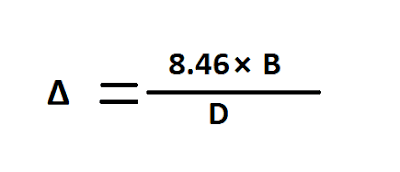Relation Between BASE, DELTA and DUTY - Civil Engineering

Relation Between Base, Delta and Duty

Let assume,
• 'D' is the duty of water, unit hectares/cumec
• 'B' is the base period of the crop, the unit in day.
• 'Δ' is the total depth of water, unit meter.
We know, one cumec of water supplying continuously for B days to obtain a depth of water Δ over an area of land D hectares.
Hence, we can write
⇒ 1 cumec for B days gives Δ over D hectares.
⇒ 1 cumec for 1 day gives Δ over D/B hectares.
⇒ 1 cumec for 1 day(1 cumec-day) = (D/B)⨯Δ hectares-meter [Equation 1].

Now, calculate the value of 1 cumec-day = 1246060=86400 m³.

We know that 1 hectare is equal to 10000 m², now convert 86400 m³ into a hectares-meter.

So, 1 cumec-day =86400 m³ = 8.64 hectares-meter [Equation 2].
From equation 1 and 2 we can writeRelation Between Base, Delta and Duty
⇰ (D/B)⨯ Δ = 8.64.
⇰ (D/B)⨯ Δ = [(8.46⨯ B)/DUnit in meter